﻿ 管路低噪声排水装置设计及试验研究
 舰船科学技术2017, Vol. 39Issue (1): 90-95PDF

1. 海军驻中国舰船研究设计中心军事代表室，湖北 武汉 430064;
2. 海军工程大学 动力工程学院，湖北 武汉 430033;
3. 中国人民解放军 91315 部队，辽宁 大连，116041

Design and testing study of low noise drainage device for pipelines
XIA Ji1, ZHANG Yang-yang2, PAN Qing-bin3
1. Naval Military Representative Office in China Ship Development and Design Center, Wuhan 430064, China;
2. Naval University of Engineering, Institute of Power Engineering, Wuhan 430033, China;
3. No. 91315 Unit of PLA, Dalian 116041, China
Abstract: Pipeline noise is one of the important source of low frequency noise of battleship, which has serious impact on the acoustic stealth performance. Aimed at typical centrifugal pump, design a low noise drainage device, which combines accumulator and Herm hertz muffler. Build simulation model by software AMESim, and verify the result by experiment. Simulation and experiment results show that low noise drainage device can effectively attenuation the pressure pulsation of pipeline of centrifugal pump, so as to release the fluid noise and pipeline vibration.
Key words: low noise drainage device     pressure pulsation     fluid noise     attenuation

1 低噪声排水装置结构及工作原理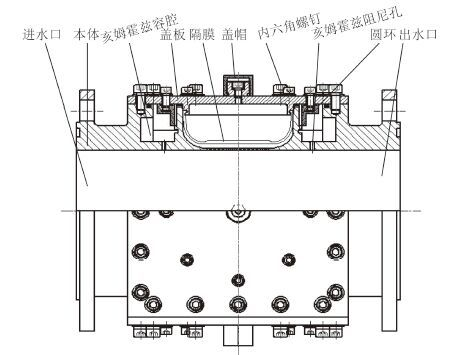图 1 低噪声排水装置结构简图 Fig. 1 Structure diagram of low noise drainage device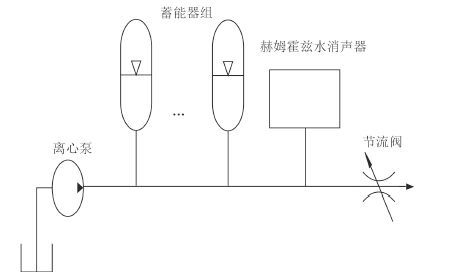图 2 低噪声排水装置的工作原理 Fig. 2 Working principle of low noise drainage device

2 结构参数优化 2.1 蓄能器参数确定

1） 蓄能器容积计算

 ${\rm{\Delta }}V = 5\% {V_p}\text{，}$ (1)

 $V = \frac{{{\rm{\Delta }}V}}{{1-{{(\frac{{2-\delta }}{{2 + \delta }})}^{1/k}}}}\text{。}$ (2)

 ${V_1} = {V_2} = {V_3} = \frac{V}{{0.9}}\text{。}$ (3)

2） 蓄能器进水口参数优化

 ${f_{{\rm{AN}}}} = \frac{{\rm{1}}}{{2{\rm{\pi }}}}\sqrt {\frac{{K{a_{\rm{A}}}{p_0}}}{{\rho {l_{\rm{A}}}{V_0}}}} \text{。}$ (4)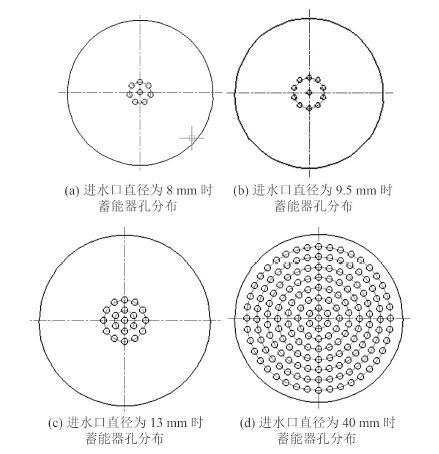图 3 蓄能器孔分布示意图 Fig. 3 Distribution of accumulator holes
2.2 亥姆霍兹水消声器参数确定

1）亥姆霍兹水消声器进水口参数确定

 ${f_{{HN}}} = \frac{{\rm{1}}}{{2{\rm{\pi }}}}\sqrt {\frac{{{\beta _{\rm{e}}}{a_{H}}}}{{\rho {l_{H}}{V_{H}}}}} \text{。}$ (5)

2） 低噪声排水装置最终结构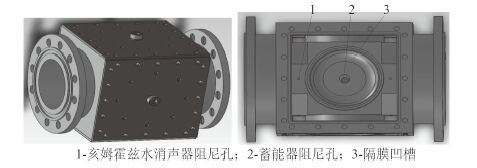图 4 低噪声排水装置结构三维示意图 Fig. 4 3D diagram of low noise drainage device
3 仿真模型的建立及仿真分析 3.1 仿真模型建立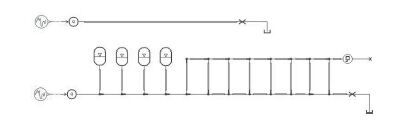图 5 低噪声排水装置 AMESim 仿真模型 Fig. 5 AMESim model of low noise drainage device

3.2 仿真分析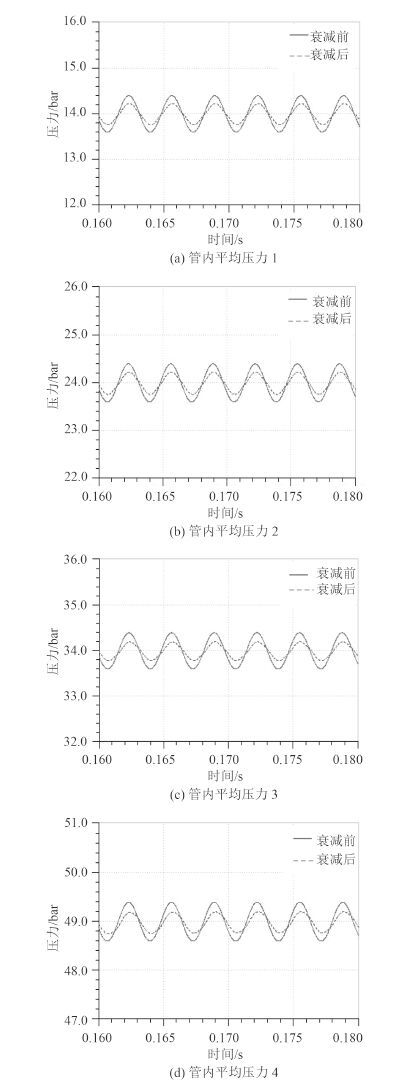图 6 低噪声排水装置衰减轴频脉动效果 Fig. 6 Axial pulsation attenuation of low noise drainage device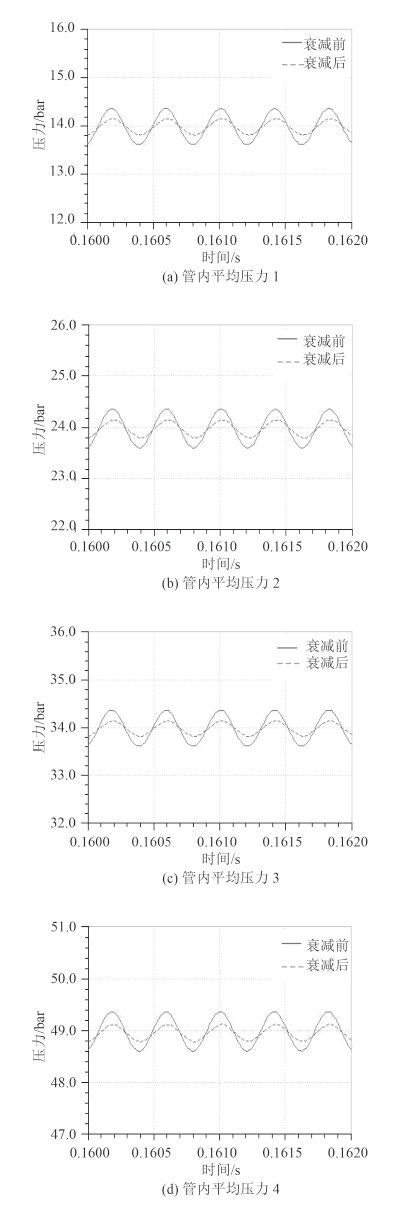图 7 低噪声排水装置衰减叶频脉动效果 Fig. 7 Blade frequency pulsation attenuation of low noise drainage device表 1 低噪声排水装置衰减轴频脉动效果 Tab.1 Axial pulsation attenuation of low noise drainage device表 2 低噪声排水装置衰减叶频脉动效果 Tab.2 Blade frequency pulsation attenuation of low noise drainage device
 $\left\{ {\begin{array}{*{20}{l}} {{\delta _{{\rm{inp}}}} = \displaystyle\frac{{{p_{{\rm{inmax}}}}-{p_{{\rm{inmin}}}}}}{{{p_{{\rm{in}}}}}},{\delta _{{\rm{outp}}}} = \displaystyle\frac{{{p_{{\rm{outmax}}}}-{p_{{\rm{outmin}}}}}}{{{p_{{\rm{out}}}}}}\text{，}}\\ {\lambda =\displaystyle \frac{{{\delta _{{\rm{inp}}}}-{\delta _{{\rm{outp}}}}}}{{{\delta _{{\rm{inp}}}}}} \times 100\% \text{，}}\\ {\Delta {L_{\rm{p}}}{\rm{ = }}20\lg \frac{{{\delta _{{\rm{inp}}}}}}{{{\delta _{{\rm{outp}}}}}}\text{。}} \end{array}} \right.$ (6)

4 实验研究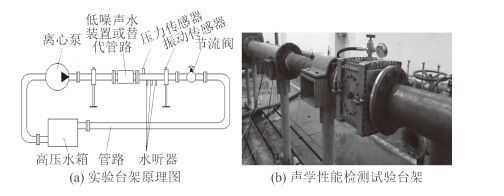图 8 声学性能检测试验台架原理及实物图 Fig. 8 Principle and physical diagram of acoustic performance test bench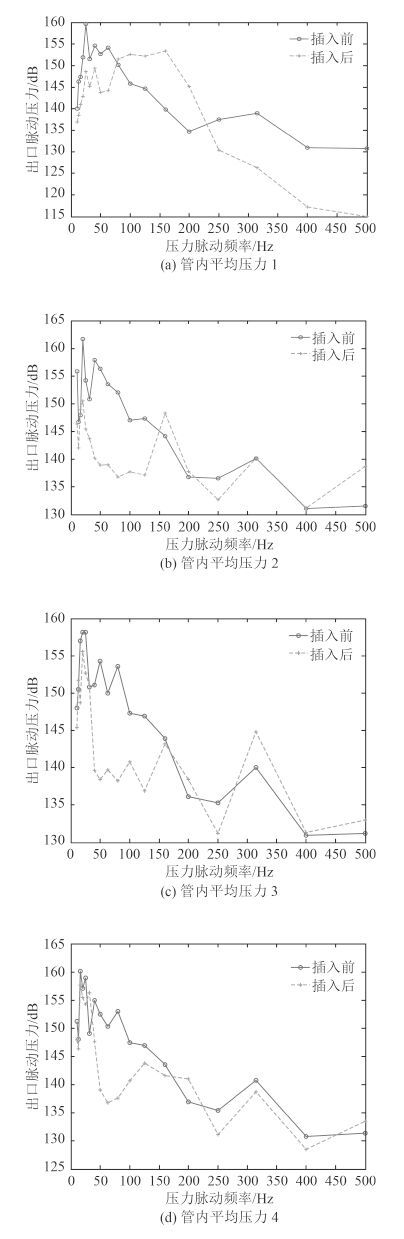图 9 低噪声排水装置出口的脉动压力 Fig. 9 Pulsation pressure of low noise drainage device exit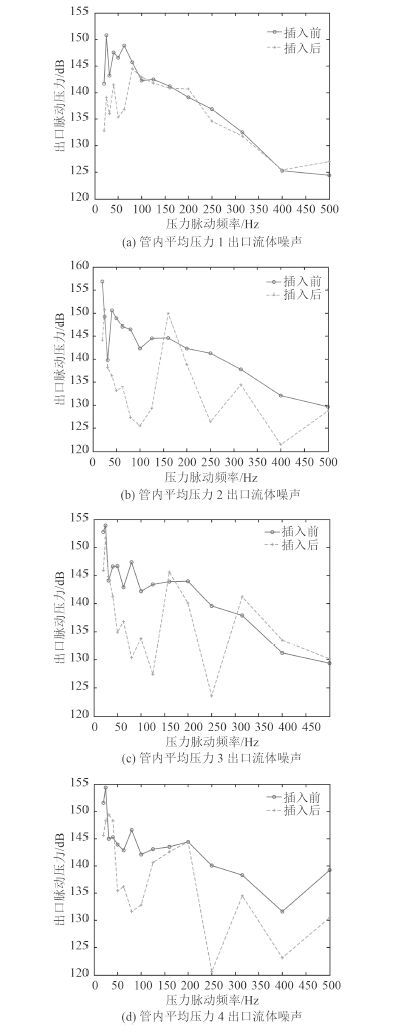图 10 低噪声排水装置出口的流体噪声 Fig. 10 Fluid noise of low noise drainage device exit

5 结 语

  赵大为, 罗小辉, 杨珍. 一种管路压力脉动抑制装置优化设计及试验分析[J]. 噪声与振动控制, 2014, 34 (6):188–191.  胡跃华, 钱文臣. 管系振动问题的分析及消振处理措施[J]. 石油化工设计技术, 2008, 25 (1):22–28.  彭利坤, 张阳阳. 舰船纵倾均衡水舱加气调水时管路冲击特性研究[J]. 液压与气动, 2013 (8):9–12.  蔡标华, 丁炜, 余健, 等. 船用舱底泵系统压力脉动抑制研究[J]. 舰船科学技术, 2012, 34 (3):65–67.  LUO X, SHI Z, HU J. Semi-active accumulator absorbing pressure pulsation in high-pressure water-jet propulsion system, Pro. instn mech. Engrs, part C:[J]. Mechanical Engineering Science, 2011 (225):2052–2061.  尹志勇, 钟荣, 刘忠族. 管路系统振动噪声控制技术研究现状与展望[J]. 舰船科学技术, 2006, 28 (S2):23–29.  窦雨淋, 张涛. 舰船管路流体脉动衰减器的性能研究[J]. 中国舰船研究, 2008, 3 (4):40–44.  CHUN M H, YU S O. A parametric study and a guide chart to avoid condensation-included water hammer in a horizontal pipe[J]. Nuclear Engineering and Design, 2000, 201 (2-3):239–257. DOI: 10.1016/S0029-5493(00)00280-6  ZHU Mar-lin, ZHANG Xiao-hong. Study on water hammer prevention in pumping water supply systems by multi-valves[J]. International Conference on Hybrid Information Technology, 2006 :342–346.